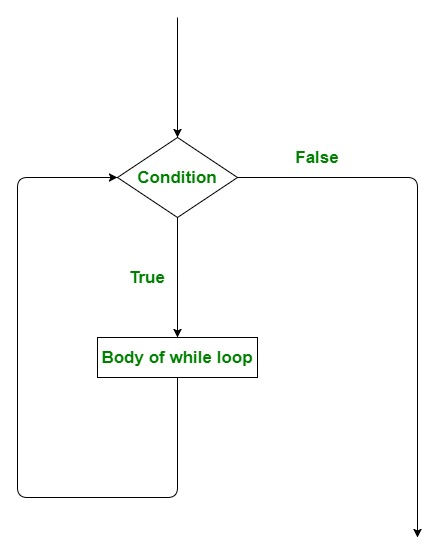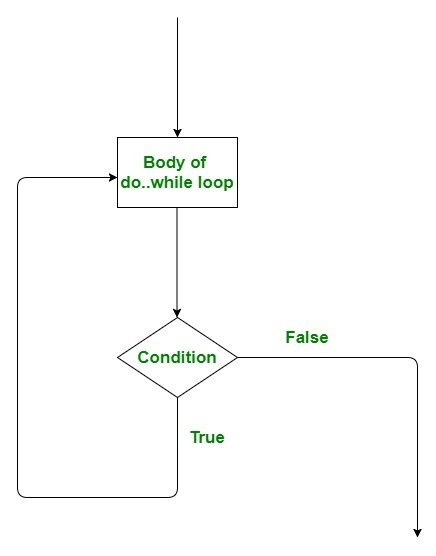# while and do while Loop in Scala

• Last Updated : 11 Aug, 2021

Looping in programming languages is a feature which facilitates the execution of a set of instructions/functions repeatedly while some condition evaluates to true. Loops make the programmers task simpler. Scala provides the different types of loops but in this article we understand while and do-while loops.

while loop

While programming there might be situation which we need to repeat until and unless a condition is met. In these cases, while loop is used. A while loop generally takes a condition in parenthesis. If the condition is True then the code within the body of the while loop is executed. A while loop is used when we don’t know the number of times we want the loop to be executed however we know the termination condition of the loop. The condition at which loop stops is called breaking condition.
Syntax:

```while (condition)
{
// Code to be executed
}```

Flowchart:Example : Execution of While loop

## Scala

 `// Scala program of while loop` `// Creating object``object` `GFG``{``    ``// Main method``    ``def` `main(args``:` `Array[String])``    ``{``        ``// variable declaration (assigning 5 to a)``        ``var` `a ``=` `5` `        ``// loop execution``        ``while` `(a ``>` `0``)``        ``{``            ``println(``"a is : "` `+ a)``            ``a ``=` `a - ``1``;``        ``}``    ``}``}`

Output:

```a is : 5
a is : 4
a is : 3
a is : 2
a is : 1```

Example : Finding element in an Array

## Scala

 `// Scala program of while loop` `// Creating object``object` `GFG``{``   ``// Main method``   ``def` `main(args``:` `Array[String])``   ``{``       ``// variable declaration (assigning 5 to a)``       ``var` `a ``=` `Array(``"do_while"``, ``"for"``, ``"while"``)``       ``var` `index ``=` `0``       ` `       ``// loop execution``       ``while` `(index ``<` `a.length)``       ``{``           ``if``(a(index) ``==` `"while"``)``               ``println(``"index of while is "` `+ index)``           ``index ``=` `index + ``1``       ``}``   ``}``}`

Output:

`index of while is 2`

Note: To execute following commands use Intellij. save this program in format file_name.scala and run it using scala in Intellij.

do while loop

A do..while loop is almost same as a while loop. The only difference is that do..while loop runs at least one time. The condition is checked after the first execution. A do..while loop is used when we want the loop to run at least one time. It is also known as the exit controlled loop as the condition is checked after executing the loop. In while loop condition is placed at top of loop Whereas in do while loop condition is placed at end, due to this positioning of condition all statements under do while gets executes at least once.
Syntax:

```do {

// statements to be Executed

} while(condition);```

Flowchart:Example : Execution of do while loop

## Scala

 `// Scala program of do-while loop` `// Creating object``object` `GFG``{``    ``// Main method``    ``def` `main(args``:` `Array[String])``    ``{``        ``// variable declaration (assigning 5 to a)``        ``var` `a ``=` `5``;` `        ``// loop execution``        ``do``        ``{``            ``println(``"a is : "` `+ a);``            ``a ``=` `a - ``1``;``        ``}``        ``while` `(a ``>` `0``);``    ``}``}`

Output:

```a is : 5
a is : 4
a is : 3
a is : 2
a is : 1```

Example : Running loop until we encounter a string in Array

## Scala

 `// Scala program for do-while loop` `// Creating object``object` `GFG``{``    ``// Main method``    ``def` `main(args``:` `Array[String])``    ``{``        ``// Declaring an array``        ``var` `a ``=` `Array(``"hello"``, ``"This"``, ``"is"``, ``"geeksforgeeks"``, ``"bye"``)``        ``var` `str ``=` `"bye"``        ``var` `i ``=` `0``        ` `        ``// loop execution``        ``do``        ``{``            ``println(``"program is saying "` `+ a(i));``            ``i ``=` `i + ``1``;``        ``}``        ``while` `(a(i) !``=` `str);``    ``}``}`

Output:

```program is saying hello
program is saying This
program is saying is
program is saying geeksforgeeks```

In above code, bye won’t be printed.

My Personal Notes arrow_drop_up